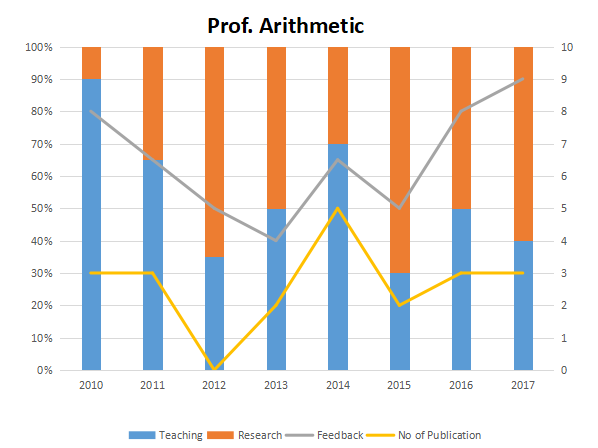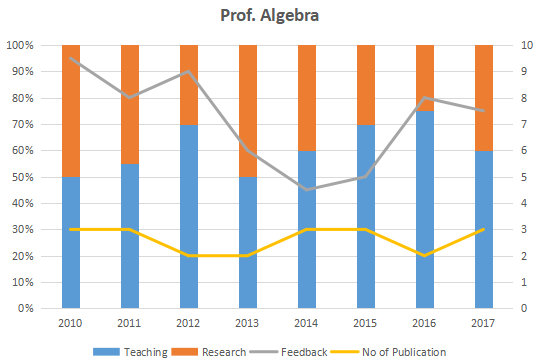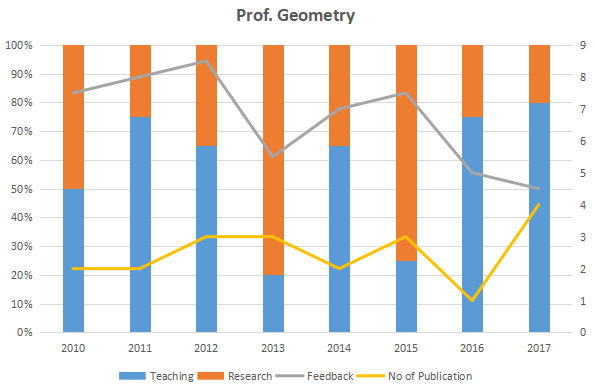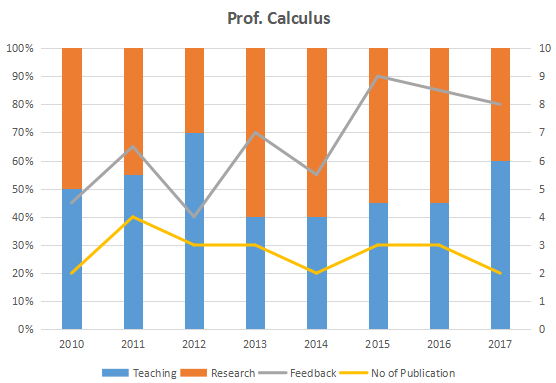### XAT 2018 Question 73

Instructions

The graph below represent the performance of four professors, across years. measured on four variables: Percentage of time spent on research, teaching, feedback on (a scale of 10. right-hand side) and number of publications (right-hand side). Assume that the cumulative time spent per year on research and teaching activities are sane for all four professors and each of them taught only one course of 90 classroom hours.Question 73

# Count the number of instances in which "annual decreasing efforts in research" is accompanied with "annual increase in feedback"?

Solution

Prof. Arithmetic: There are two such instances, during 2013-14 and 2015-16

Prof. Algebra: There are three such instances, during 2011-12, 2014-15 and 2015-16

Prof. Geometry: There are two such instances, during 2010-11 and 2013-14

Prof. Calculus: There are two such instances, during 2010-11 and 2014-15

Thus, there are 2+3+2+2 = 9 such instances.

Hence, option C is the answer.